# Facewise brain cortical surface area: scripts now availableIn the paper Measuring and comparing brain cortical surface area and other areal quantities (Neuroimage, 2012;61(4):1428-43, doi:10.1016/j.neuroimage.2012.03.026, pmid:22446492), we described the steps to perform pycnophylactic interpolation, i.e., mass-conservative interpolation, of brain surface area. The method is also valid for any other quantity that is also areal by nature.

The scripts to perform the steps we propose in the paper are now freely available for Octave and/or matlab. They can also be executed directly from the shell using Octave as the interpreter. In this case, multiple instances can be easily submitted to run in a cluster. Click here to dowload and for step-by-step instructions.

# Retrospective motion correction for structural MRI

Good quality results can only be obtained with good quality images. To obtain images with good SNR and high resolution, long scan times are often necessary. However, with longer acquisition, the subjects are more likely to move. Rather than acquire a very long sequence, that often can last up to 40 minutes, the acquisition can be broken into many shorter scans, each with lower SNR. These are coregistered, effectively reducing the effect of undesired movement, and averaged, for increase of the SNR (Kochunov et al, 2006).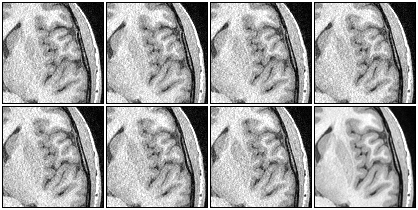The last image in the second row is the average of all the other seven, after retrospective motion correction has been applied.

The results above are typical. Each pixel above corresponds to a true voxel, with no interpolation. Therefore, to see in more detail, simply download the image above and use an image viewer (e.g. gwenview or eog) to zoom.

The method is termed retrospective to discern it from prospective motion correction methods, such as those available for BOLD-sensitive EPI acquisitions (e.g. Siemens 3D PACE).

Since the images belong to the same subject and typically the movement is of only a few degrees or milimeters, the affine matrices for these corrections should have a diagonal with values very close to 1 and very close to zero off the diagonal. Here is one example:

0.999995    -0.00020007  -0.0030414    0.106906
0.000188555  0.999993    -0.00378601   0.100499
0.00304214   0.00378542   0.999988    -0.204133
0            0            0            1

As usual, the improvement in the SNR is given by a factor of$\sqrt{n}$, where$n$ is the number of images averaged.

## Download

A script to apply the method is available here: stereomc. Just download and make it executable. Call it without arguments to obtain usage information.

## Requirements

FSL tools must be installed and properly configured.

## Reference

Kochunov P, Lancaster JL, Glahn DC, Purdy D, Laird AR, Gao F, Fox P. Retrospective motion correction protocol for high-resolution anatomical MRI. Hum Brain Mapp. 2006 Dec;27(12):957-62.

# Importing FreeSurfer subcortical structures into Blender

In a previous article, a method to import cortical meshes from FreeSurfer into Blender and other CG applications was presented. Here it is complemented with a method to import the subcortical structures. These structures are segmented as part of FreeSurfer subcortical stream, and are stored in a volume-based representation. In order to import into Blender, first it is necessary to generate surfaces, then convert to Wavefront OBJ, and finally, do the import. Below is an example of how the output looks like.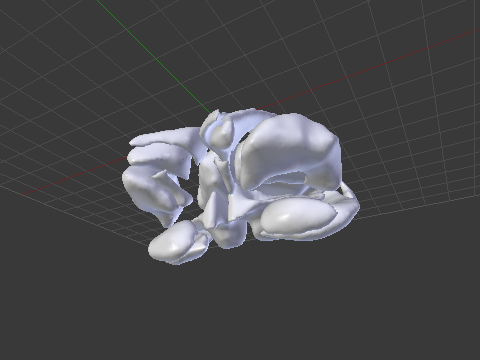An example of a subcortical meshes generated from FreeSurfer subcortical segmentations imported into Blender.

## Generating the surfaces for subcortical structures

The surfaces can be generated using FreeSurfer’s own tools. The script aseg2srf automates the task. Suppose you would like to create meshes for all subcortical structures of the subjects named “capitu”, “bentinho” and “ezequiel”. Simply run:

aseg2srf -s "capitu bentinho ezequiel"


# Importing FreeSurfer cortical meshes into Blender

Computer graphics (CG) applications, such as Blender, Maya or 3D Studio, doesn’t come with filters that allow to directly import or export FreeSurfer meshes. To import brain surfaces to these applications, converting first to an intermediate file format solves the problem. Below is an example of a brain imported into Blender.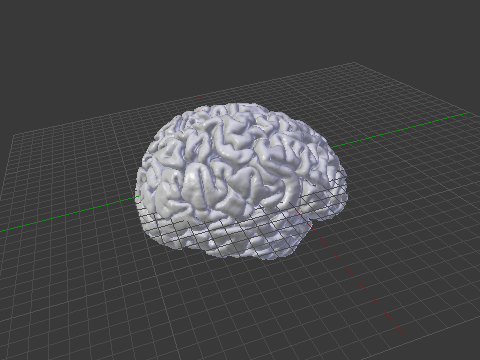An example of a brain mesh from FreeSurfer imported into Blender after conversion to Wavefront OBJ.

The mris_convert, from FreeSurfer, allows conversion to stereolithography format (STL). However, not all CG applications read natively from STL files. Moreover, other formats, such as Wavefront OBJ and Stanford PLY have simpler structure — yet allowing features that will be discussed here in forthcoming articles.

## Conversion from FreeSurfer to Wavefront OBJ

A simple procedure to convert a FreeSurfer surface to Wavefront OBJ (ASCII) is:

1) Use mris_convert to convert from FreeSurfer binary to ASCII:

mris_convert lh.pial lh.pial.asc


2) A suggestion is to rename the .asc file to .srf, to differentiate it from other files that also have .asc extension and have a different internal structure.

mv lh.pial.asc lh.pial.srf


3) Use the script srf2obj to convert from .srf to .asc:

srf2obj lh.pial.srf > lh.pial.obj


The symbol “>” is necessary so that the output from srf2obj is redirected to a new file.

## Conversion from Wavefront OBJ to FreeSurfer

To convert back to FreeSurfer, a similar strategy is used.

1) Use the script obj2srf to generate new .srf files (here directly named as .asc, as expected by mris_convert)

obj2srf lh.pial.obj > lh.pial.asc


2) Use mris_convert:

mris_convert lh.pial.asc lh.pial


## Download

Both scripts are available here: srf2obj and obj2srf. Right-click and save, then make them executable.

## Requirements

The scripts use gawk as the interpreter, and expects to find it on its usual location (/bin/gawk). If in your system gawk is installed elsewhere, modify the first line accordingly.

If you don’t have gawk, you can get it from the repository of your Linux distribution. For Mac, gawk is available in MacPorts and Fink. Alternatively, you may replace gawk with awk itself, nawk, or any other awk implementation that works.

# Confidence intervals for Bernoulli trials

A Bernoulli trial is an experiment in which the outcome can be one of two mutually exclusive results, e.g. true/false, success/failure, heads/tails and so on. A number of methods are available to compute confidence intervals after many such trials have been performed. The most common have been discussed and reviewed by Brown et al. (2001), and are presented below. Consider$n$ trials, with$X$ successes and a significance level of$\alpha=0.05$ to obtain a 95% confidence interval. For each of the methods, the interval is shown graphically for$1 \leqslant n \leqslant 100$ and$1 \leqslant X \leqslant n$.

## Wald

This is the most common method, discussed in many textbooks, and probably the most problematic for small samples. It is based on a normal approximation to the binomial distribution, and it is often called “Wald interval” for it’s relationship with the Wald test. The interval is calculated as:

• Lower bound:$L=p-k\sqrt{pq/n}$
• Upper bound:$U=p+k\sqrt{pq/n}$

where$k = \Phi^{-1}\{1-\alpha/2\}$,$\Phi^{-1}$ is the probit function,$p=X/n$ and$q=1-p$.

## Wilson

This interval appeared in Wilson (1927) and is defined as:

• Lower bound:$L = \tilde{p} - (k/\tilde{n})\sqrt{npq+k^2/4}$
• Upper bound:$U = \tilde{p} + (k/\tilde{n})\sqrt{npq+k^2/4}$

where$\tilde{p} = \tilde{X}/\tilde{n}$,$\tilde{n}=n+k^2$,$\tilde{X} = X+ k^2/2$, and the remaining are as above. This is probably the most appropriate for the majority of situations.

## Agresti-Coull

This interval appeared in Agresti and Coull (1998) and shares many features with the Wilson interval. It is defined as:

• Lower bound:$L = \tilde{p} - k\sqrt{\tilde{p}\tilde{q}/\tilde{n}}$
• Upper bound:$U = \tilde{p} + k\sqrt{\tilde{p}\tilde{q}/\tilde{n}}$

where$\tilde{q}=1-\tilde{p}$, and the remaining are as above.

## Jeffreys

This interval has a Bayesian motivation and uses the Jeffreys prior (Jeffreys, 1946). It seems to have been introduced by Brown et al. (2001). It is defined as:

• Lower bound:$L = \mathcal{B}^{-1}\{\alpha/2,X+1/2,n-X+1/2\}$
• Upper bound:$U = \mathcal{B}^{-1}\{1-\alpha/2,X+1/2,n-X+1/2\}$

where$\mathcal{B}^{-1}\{x,s_1,s_2\}$ is the inverse cdf of the beta distribution (not to be confused with the beta function), at the quantile$x$, and with shape parameters$s_1$ and$s_2$.

## Clopper-Pearson

This interval was proposed by Clopper and Pearson (1934) and is based on a binomial test, rather than on approximations, hence sometimes being called “exact”, although it is not “exact” in the common sense. It is considered overly conservative.

• Lower bound:$L = \mathcal{B}^{-1}\{\alpha/2,X,n-X+1\}$
• Upper bound:$U = \mathcal{B}^{-1}\{1-\alpha/2,X+1,n-X\}$

where$\mathcal{B}^{-1}\{x,s_1,s_2\}$ is the inverse cdf of the beta distribution as above.

## Arc-Sine

This interval is based on the arc-sine variance-stabilising transformation. The interval is defined as:

• Lower bound:$L = \sin\{\arcsin\{\sqrt{a}\} - k/(2\sqrt{n})\}^2$
• Upper bound:$U = \sin\{\arcsin\{\sqrt{a}\} + k/(2\sqrt{n})\}^2$

where$a=\frac{X+3/8}{n+3/4}$ replaces what otherwise would be$p$ (Anscombe, 1948).

## Logit

This interval is based on the Wald interval for$\lambda = \ln\{\frac{X}{n-X}\}$. It is defined as:

• Lower bound:$L = e^{\lambda_L}/(1+e^{\lambda_L})$
• Upper bound:$U = e^{\lambda_U}/(1+e^{\lambda_U})$

where$\lambda_L = \lambda - k\sqrt{\hat{V}}$,$\lambda_U = \lambda + k\sqrt{\hat{V}}$, and$\hat{V} = \frac{n}{X(n-X)}$.

## Anscombe

This interval was proposed by Anscombe (1956) and is based on the logit interval:

• Lower bound:$L = e^{\lambda_L}/(1+e^{\lambda_L})$
• Upper bound:$U = e^{\lambda_U}/(1+e^{\lambda_U})$

The difference is that$\lambda=\ln\{\frac{X+1/2}{n-X+1/2}\}$ and$\hat{V}=\frac{(n+1)(n+2)}{n(X+1)(n-X+1)}$. The values for$\lambda_L$ and$\lambda_U$ are as above.

## Octave/MATLAB implementation

A function that computes these intervals is available here: confint.m.

# Braindering with ASCII files

Binary file formats are faster to read and occupy less space for storage. However, when developing tools or when analysing data using non-standard methods, ascii files are easier to treat, and allow certain errors to be spotted immediately. Working with ascii files offer a number of advantages in these circumstances: the content of the files are human readable, meaning that there is no need for any special program other than perhaps a simple text editor, and can be manipulated easily with tools as sed or awk. It should be noted though, that while binary files require attention with respect to endianess and need some metadata to be correctly read, ascii need proper attention to end-of-line characters, that vary according to the operating system.

In articles to be posted soon I hope to share some tools to analyse and visualise brain imaging data. To use these tools, the files must be in ascii format. Each of these formats is discussed below and an example is given for a simple octahedron.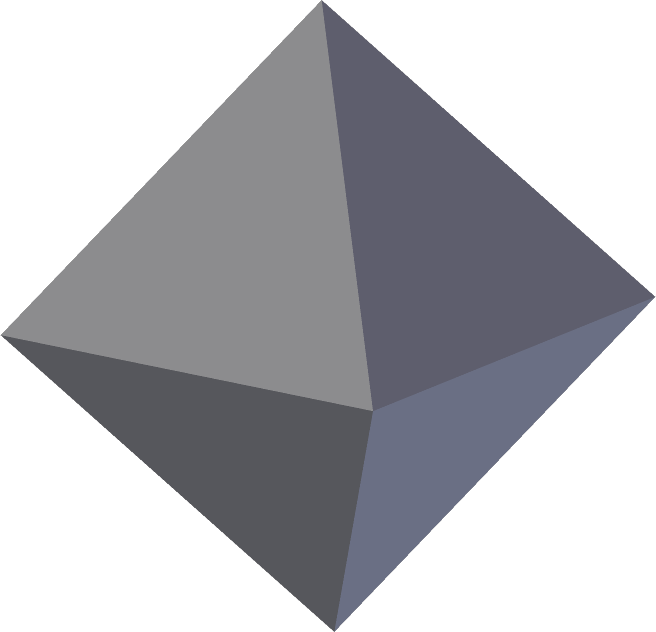## FreeSurfer surface (*.asc | suggested: *.srf)

FreeSurfer natively saves its surface files in binary form. The command mris_convert allows conversion to ascii format. The file extension is *.asc. This extension, however, is ambiguous with the curvature format (see below), reason for which a recommendation is to rename from *.asc to *.srf immediately after conversion.

The *.asc/*.srf format has a structure as described below. This format does not allow storing normals, colors, materials, multiple objects or textures. Just simple geometry.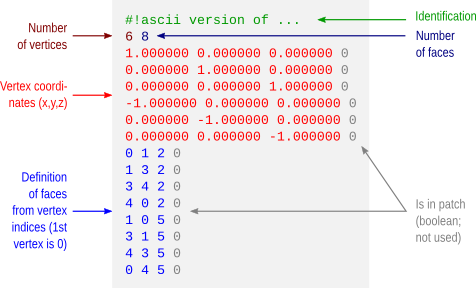## FreeSurfer curvature (*.asc | suggested: *.dpv)

A curvature file contains a scalar value assigned to each vertex. This file format does not store the geometry of the surface and, in binary form, a curvature file simply contains one scalar per vertex. In ascii format, the vertex coordinates are also saved, but not how these vertices relate to define faces and the overall geometry.

Like with the FreeSurfer surface files, curvature files in ascii format also have the extension *.asc. To avoid confusion, it is suggested to rename from *.asc to *.dpv (data-per-vertex) immediately after conversion.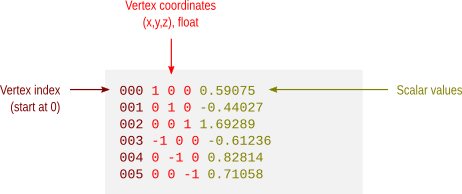## Facewise data (*.dpf)

This is not a native format from FreeSurfer, but a modification over the ascii curvature file described above. The file stores a scalar value per face, i.e. data-per-face (dpf) and, instead of saving vertex coordinates as with the *.dpv files, here the vertex indices that define the faces are saved. It is done like so because if both *.dpv and *.dpf files are present for a given geometric object, the object can be reconstructed with very little manipulation using simply awk. Currently there is no binary equivalent format.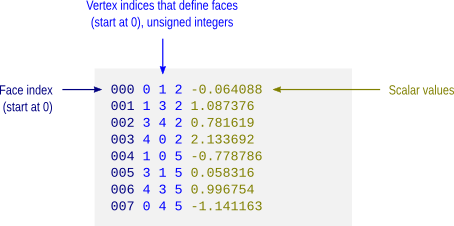## VTK polygonal data (*.vtk)

A number of file formats have been developed over the years for use with the Visualization Toolkit (vtk). Some of these formats are now considered as “legacy” and are being phased out in favour of xml formats. Despite this, they are still used by a number of applications, such as fsl/first. The octahedron above in vtk polygonal data (polydata) format would have a structure as depicted below.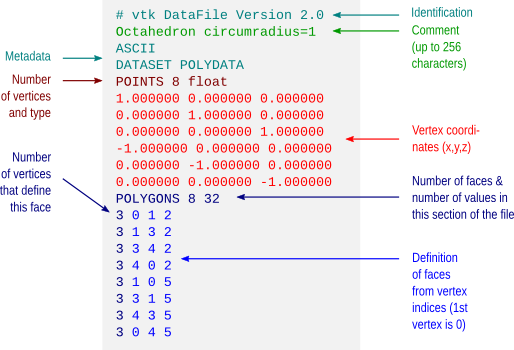## Wavefront Object (*.obj)

Wavefront Object files have a very simple structure, yet are powerful to allow definition of textures, normals and different materials. The format is supported by a variety of different graphic applications. The octahedron above would have a file structure as shown below.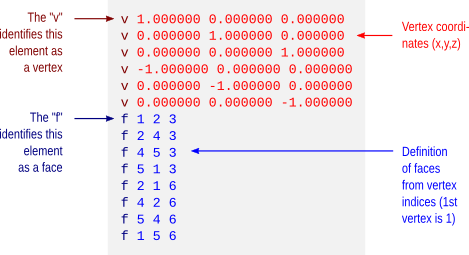The example does not show colors. However, it is possible to assign a material to each face, and materials contain their own color. These materials are typically stored in a separate file, a Materials Library, with extension *.mtl. An example for the same octahedron, both the object file and its associated materials library, is here: octa-color.obj and octa-color.mtl.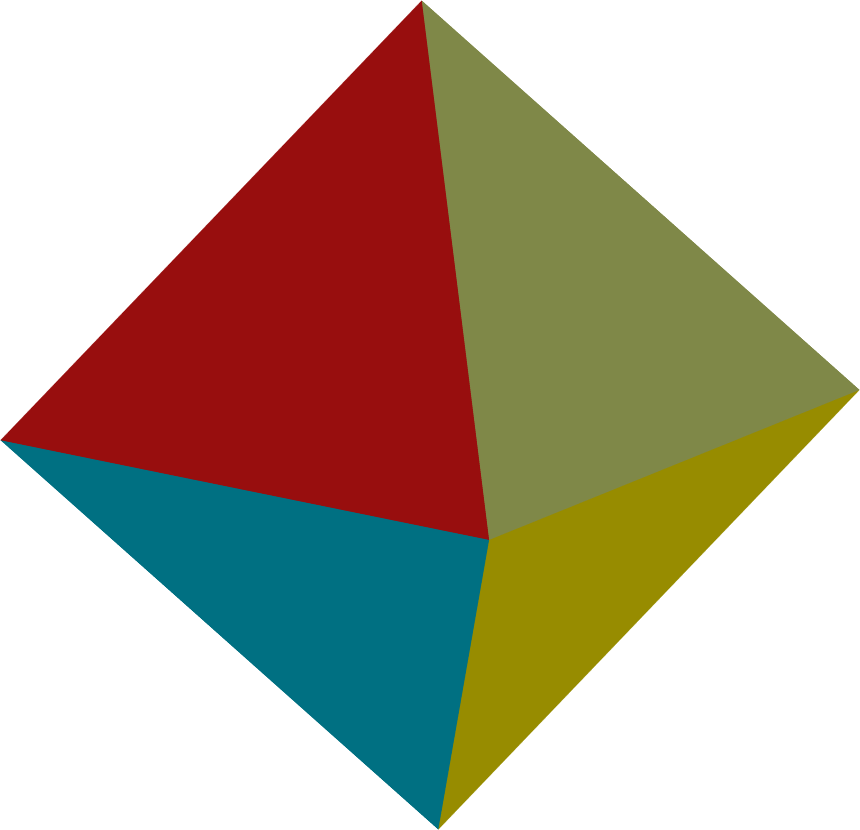Many computer graphics software, however, limit the number of colors per object to a relatively small number, as 16 or 32. Blender, however, allows 32767 colors per object, a comfortable space for visualization of complex images, such as brain maps.

## Stanford Polygon (*.ply)

The Stanford Polygon file allows not only the geometry to be stored, but also allow colours, transparency, confidence intervals and other properties to be assigned at each vertex. Again, the octahedron would have a file structure as: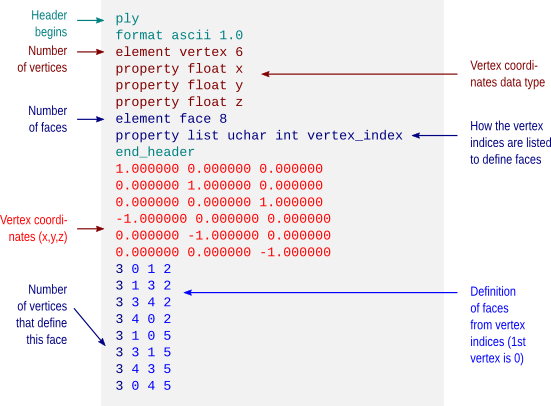This format also allows color attributes to be assigned to each vertex, as shown below.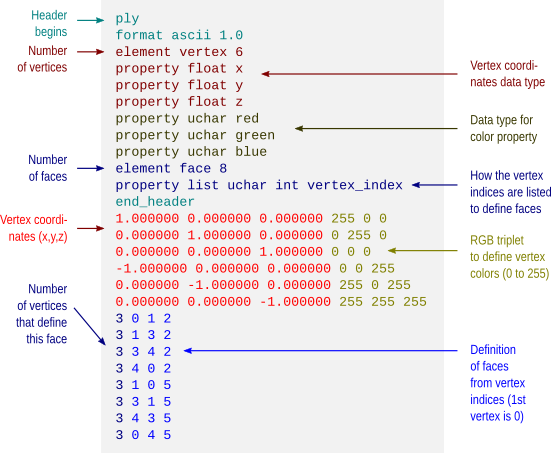The resulting object would then look like: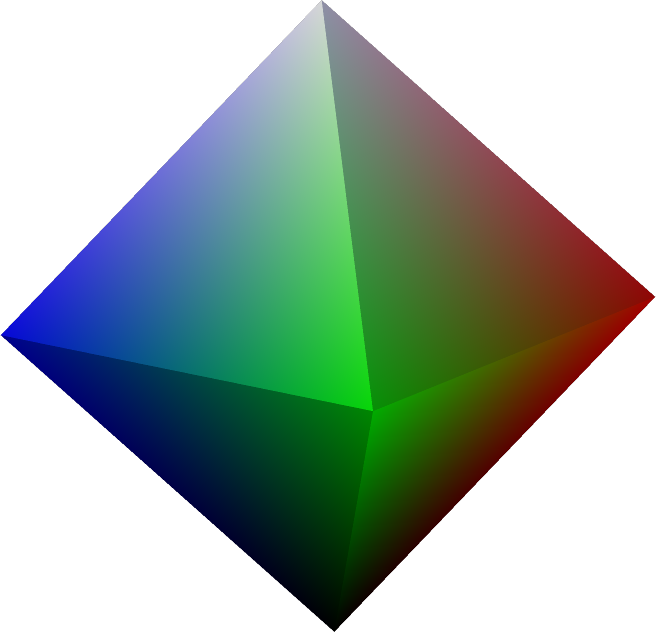## Batch conversion

Conversion between FreeSurfer formats can be done using mris_convert. A script to batch convert all FreeSurfer surface and curvature files from binary format to ascii is available here: subj2ascii. Before running, make sure FreeSurfer is correctly configured, that the variable SUBJECTS_DIR has been correctly set, and that you have writing permissions to this directory. Execute the script with no arguments to get usage information. The outputs (*.srf and *.dpv) are saved in a directory called ascii inside the directory of the subject. For many subjects, use a for-loop in the shell.

## For more information

• A description of the FreeSurfer file formats is available here.
• A description of the different vtk (legacy and current) is available here.
• Specification of the Wavefront obj format is here. Material library files are described here.
• A description of the Stanford ply format is here.

Update — 28.May.2012: A script to convert from FreeSurfer .asc/.srf to .obj is here.
Update — 26.Jul.2013: This post was just updated to include examples of color formats.

# Quickly inspect FreeSurfer cortical surfaces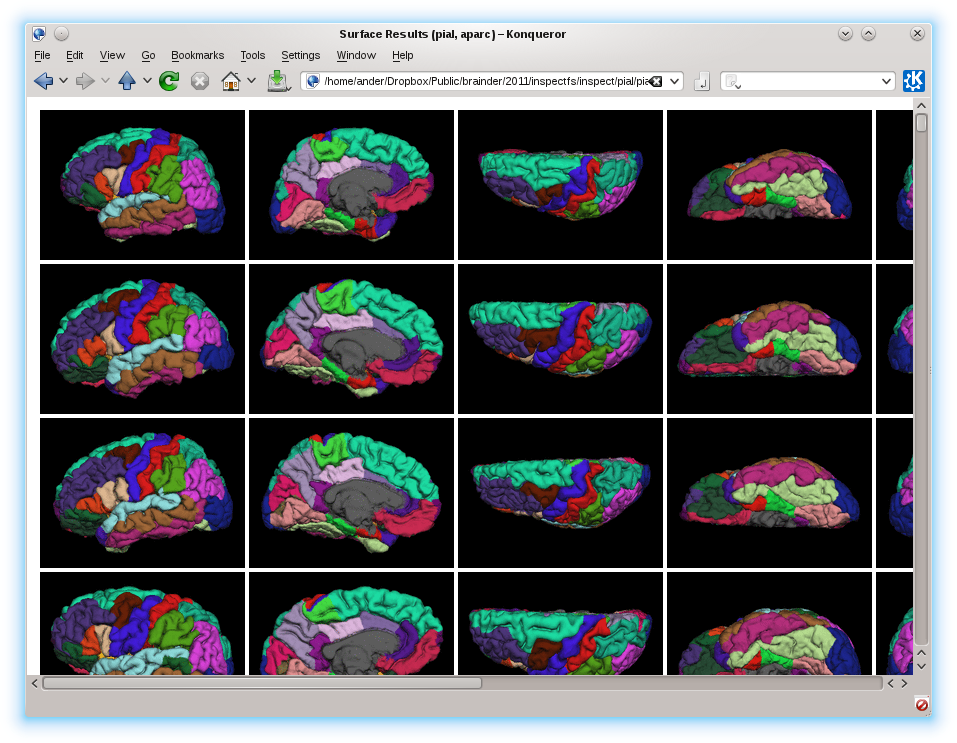To quickly inspect the surface reconstruction and parcellations produced by the cortical stream of FreeSurfer, rather than laboriously open each hemisphere at a time in tksurfer, an easier solution is to run a script that captures images, organise and present them all in a single html page that can be seen in any browser.

## Before you start

Before you start, make sure you have:

• FreeSurfer, any version released in the last 2 years. The procedure has been tested up to version 5.1.0.
• ImageMagick, any recent version. For most Linux, ImageMagick can be installed directly from the distro’s repository. For Mac, it can be obtained with MacPorts or Fink.
• The scripts takeshots, makehtml, shots_tksurfer.tcl, and shots_tkmedit.tcl. Put all these scripts into the same directory and make takeshots and makehtml executable.

## Capturing the images

1. Make sure recon-all finished for all your subjects. If there are no results, obviously there won’t be anything to inspect.
2. Make sure the variable SUBJECTS_DIR is correctly set, pointing to the directory that contains the subjects, and that you have writing permissions to this directory.
3. Prepare a text file containing a list of subjects, one per line, like this:
subj001 subj002 ... subjNN
4. Run the takeshots script. A typical usage is like this:
./takeshots -l listsubj.txt -m pial -m inflated -p aparc
The options are:
-l: List of subjects.
-s: Additional subjects not in the list.
-m: Surfaces to inspect (pial, inflated, white, sphere, etc).
-p: Parcellations to inspect (aparc, aparc.a2005s, aparc.a2009s, etc).
This script will create a subdirectory called ‘shots’ inside the directory of each subject, and will store a number of .tif files that are the different views of the cortical surfaces.
5. Run the makehtml script. A typical usage is like this:
./makehtml -l listsubj.txt -m pial -m inflated -p aparc -d /path/to/html
The options are identical to the previous command, with the addition of the option -d that lets you specify a directory where the html will be created. If this directory doesn’t exist, it will be created.

## What you get

Open the file index.html in the directory specified in the previous step. There you’ll see all the surfaces of all the subjects, all in the same page, allowing you to quickly compare and have an idea of how good or bad they are. To see the name of the subject, hover the mouse on the corresponding image. Click on the image to see it larger. An example, using only pial and inflated surfaces, and the aparc parcellation, is here.

# False Discovery Rate: Corrected & Adjusted P-values

Until mid-1990’s, control of the error rate under multiple testing was done, in general, using family-wise error rate (FWER). An example of this kind of correction is the Bonferroni correction. In an influential paper, Benjamini and Hochberg (1995) introduced the concept of false discovery rate (FDR) as a way to allow inference when many tests are being conducted. Differently than FWER, which controls the probability of committing a type I error for any of a family of tests, FDR allows the researcher to tolerate a certain number of tests to be incorrectly discovered. The word rate in the FDR is in fact a misnomer, as the FDR is the proportion of discoveries that are false among all discoveries, i.e., proportion of incorrect rejections among all rejections of the null hypothesis.

## Benjamini and Hochberg’s FDR-controlling procedure

Consider testing$N$ hypotheses,$H_{1}, H_{2}, \ldots , H_{N}$ based on their respective p-values,$p_{1}, p_{2}, \ldots , p_{N}$. Consider that a fraction$q$ of discoveries are allowed (tolerated) to be false. Sort the p-values in ascending order,$p_{(1)} \leq p_{(2)} \leq \ldots \leq p_{(N)}$ and denote$H_{(i)}$ the hypothesis corresponding to$p_{(i)}$. Let$k$ be the largest$i$ for which$p_{(i)} \leq \frac{i}{N}\frac{q}{c(N)}$. Then reject all$H_{(i)}$,$i=1, 2, \ldots , k$. The constant$c(N)$ is not in the original publication, and appeared in Benjamini and Yekutieli (2001) for cases in which independence cannot be ascertained. The possible choices for the constant are$c(N)=1$ or$c(N)=\sum_{i=1}^{N} 1/i$. The second is valid in any situation, whereas the first is valid for most situations, particularly where there are no negative correlations among tests. The B&H procedure has found many applications across different fields, including neuroimaging, as introduced by Genovese et al. (2002).

## FDR correction

The procedure described above effectively defines a single number, a threshold, that can be used to declare tests as significant or not at the level$q$. One might, instead, be interested to know, for each original p-value, the minimum$q$ level in which they would still be rejected. To find this out, define$q_{(i)}=\frac{p_{(i)}N}{i}c(N)$. This formulation, however, has problems, as discussed next.

## FDR adjustment

The problem with the FDR-correction is that$q_{(i)}$ is not a monotonic function of$p_{(i)}$. This means that if someone uses any$q_{(i)}$ to threshold the set of FDR-corrected values, the result is not the same as would be obtained by applying sequentially the B&H procedure for all these corresponding$q$ levels.

To address this concern, Yekutieli and Benjamini (1999) introduced the FDR-adjustment, in which monotonicity is enforced, and which definition is compatible with the original FDR definition. Let$q*_{(i)}$ be the FDR-adjusted value for$p_{(i)}$. It’s value is the smallest$q_{(k)}, k \geq i$, where$q_{(k)}$ is the FDR-corrected as defined above. That’s just it!

## Example

Consider the image below, on the left, a square of 9×9 pixels containing each some t-statistic with some sparse, low magnitude signal added. On the right are the corresponding p-values, ranging approximately between 0-1:

Statistical maps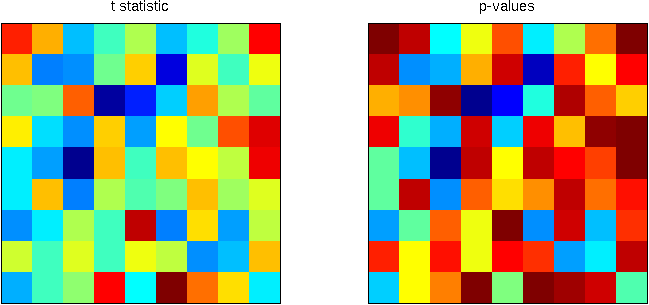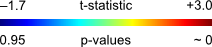Using the B&H procedure to compute the threshold, with$q=0.20$, and applying this threshold to the image rejects the null hypothesis in 6 pixels. On the right panel, the red line corresponds to the threshold. All p-values (in blue) below this line are declared significant.

FDR-threshold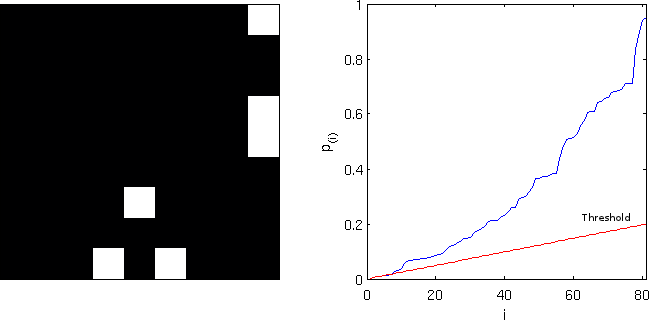Computing the FDR-corrected values at each pixel, and thresholding at the same$q=0.20$, however, does not produce the same results, and just 1 pixel is declared significant. Although conservative, the “correction” is far from the nominal false discovery rate and is not appropriate. Note on the right panel the lack of monotonicity.

FDR-corrected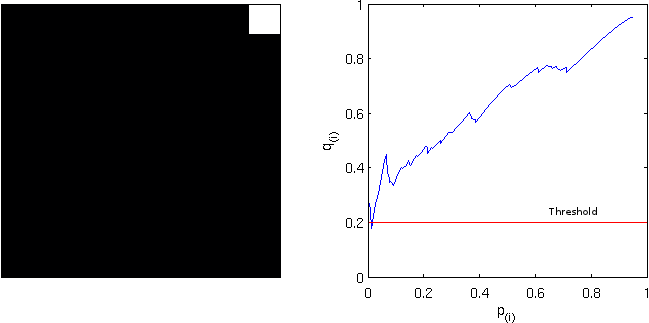Computing instead the FDR-adjusted values, and thresholding again at$q=0.20$ produces the same results as with the simpler FDR-threshold. The adjustment is, thus, more “correct” than the “corrected”. Observe, on the right panel, the monotonicity enforced.

FDR-adjusted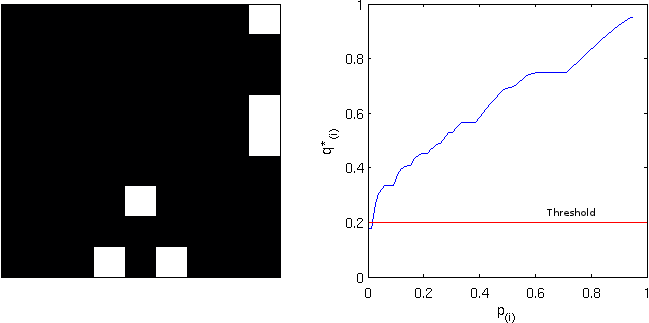## Implementation

An implementation of the three styles of FDR for Octave/MATLAB is available here: fdr.m
SPM users will find a similar feature in the function spm_P_FDR.m.

# Converting OASIS brains to NIFTI

The OASIS dataset consists of a number of T1-weighted mri brain scans, which has been kindly offered online at http://www.oasis-brains.org. The dataset can be downloaded for free after accepting an academic agreement available at the website. The data was released in analyze 7.5 file format. This format, despite having been used by the neuroimaging community for many years, suffers from not including orientation information. The nifti format addresses this concern, but when converting, some care has to be taken to ensure that there are no left-right flips. Fortunately, for this dataset, during acquisition a capsule of vitamin E was placed next to the head of each subject, on the left side, which is very helpful to identify the correct side (Marcus et al., 2007).

There are still problems, though. The nifti format has not been fully implemented in all common software packages and worse, some packages interpret differently the information contained in the header. Images that look fine in fsl‘s FSLview or FreeSurfer‘s Freeview may look stretched or shrunken in spm for instance. And images that look fine in both, may still be oriented incorrectly in Mango. Although a bit cumbersome, the procedure below ensures that the oasis images can be converted from analyze to nifti in a way that it can correctly read and shown by Mango, fsl, FreeSurfer and spm. The procedure uses exclusively fsl tools:

1. Convert to nifti:
fslchfiletype NIFTI_GZ OAS1_0001_MR1_mpr-1_anon.hdr
2. Make sure there is no undesired orientation information:
fslorient -deleteorient OAS1_0001_MR1_mpr-1_anon.nii.gz
3. Set the sform_code as 2, which is for “aligned anatomy”. Although this is still in native, not aligned space, it ensures that software will read them appropriately:
fslorient -setsformcode 2 OAS1_0001_MR1_mpr-1_anon.nii.gz
4. Set the sform as the following matrix:
fslorient -setsform  0 0 -1.25 0  1 0 0 0  0 1 0 0  0 0 0 1  OAS1_0001_MR1_mpr-1_anon.nii.gz
5. Swap the order of the data. Again, this isn’t really necessary, except to ensure that different applications will all read correctly:
fslswapdim OAS1_0001_MR1_mpr-1_anon.nii.gz RL PA IS OAS1_0001_MR1_mpr-1_anon.nii.gz
6. fsl tries to preserve orientation and, when the voxels are reordered, it modifies the header accordingly, resulting in no net transformation when seen with fsl tools. To resolve this, it’s necessary to change the header again, now the qform:
fslorient -setqform -1.25 0 0 0  0 1 0 0  0 0 1 0  0 0 0 1  OAS1_0001_MR1_mpr-1_anon.nii.gz

These steps can be placed inside a simple loop within the shell for either Linux and Mac, like below (click here to download):

#!/bin/bash

# Directory where the OASIS data is located
ROOTDIR=/Volumes/HD2/oasis-dataset-416subj/original

# Directory to save results
NIFTIDIR=${ROOTDIR}/../nifti # Go to the directory with the data cd${ROOTDIR}

# For each subject
for s in * ; do

# Some feedback in the screen
echo ${s} # Create directory to save the results, if not existing mkdir -p${NIFTIDIR}/${s} # Directory of the original, raw data cd${ROOTDIR}/${s}/RAW # For each acquisition for a in *.hdr ; do # Do each of the 6 steps described in the blog${FSLDIR}/bin/fslchfiletype NIFTI_GZ ${a}${NIFTIDIR}/${s}/${a%.hdr}
${FSLDIR}/bin/fslorient -deleteorient${NIFTIDIR}/${s}/${a%.hdr}
${FSLDIR}/bin/fslorient -setsformcode 2${NIFTIDIR}/${s}/${a%.hdr}
${FSLDIR}/bin/fslorient -setsform 0 0 -1.25 0 1 0 0 0 0 1 0 0 0 0 0 1${NIFTIDIR}/${s}/${a%.hdr}
${FSLDIR}/bin/fslswapdim${NIFTIDIR}/${s}/${a%.hdr} RL PA IS ${NIFTIDIR}/${s}/${a%.hdr}${FSLDIR}/bin/fslorient -setqform -1.25 0 0 0  0 1 0 0  0 0 1 0  0 0 0 1  ${NIFTIDIR}/${s}/${a%.hdr} done done echo "Done!"  The reference for the oasis dataset is: # FreeSurfer brains in arbitrary colours Suppose you run a statistical test for each of the regions of the parcellated brain produced by FreeSurfer. It can be either surface area comparison between groups, or maybe differences in thickness. For each region, you may obtain a statistical score and likely a p-value as well. How to display these results in a color-coded brain model, still inside FreeSurfer?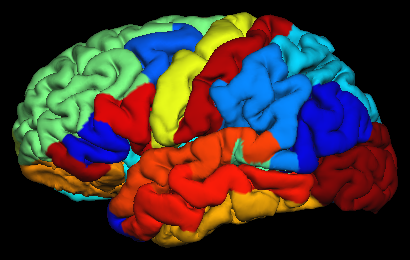The process is consists of three steps: 1. Create of a table with your results, in a format that FreeSurfer can read. 2. Embed the table in an annotation file. 3. Display in tksurfer. ## 1. Create a table The table must contain not the actual scalars, but instead rgb triplets. This can be done using a simple Octave or matlab script. Since often these results are tabulated as spreadsheets, an alternative, straightforward way is using software like LibreOffice, which gives instantaneous graphical feedback of how the resulting table looks like. An example of such a spreadsheet is this: colormap.ods. The data go into the sheets named lh.data and rh.data. For structures that are not supposed to display any color, put an off-range value below the minimum value of the other regions (e.g. -1 if the scalars are all positive). Do not add or remove structures. The sheet named colormap contains the key between the scalar values and the actual rgb colors. In this sheet, the first column (column A) is simply a rank number, used in the calculations; the second column (col. B) contains the range of values between 0 and max, regularly spaced. The remaining three columns (C, D and E) contains the rgb triplets to be assigned to each value in column B. For each scalar to be shown, the closest number has its corresponding rgb used. The sheets named lh.aparc.annot.ctab and rh.aparc.annot.ctab contain the resulting tables that will be used for actual display purposes. Each contain an index number, starting from 0, the structure name as used in FreeSurfer, the rgb colors, and a fourth value that is called “flag” and is usually zero. In most cases, only the numerical values in lh.data and rh.data have to be changed. In some occasions, it may be necessary to change the range to be shown (i.e., column B on the colormap sheet), or the colormap itself (columns C, D and E). Different colormaps can be generated, for instance, using Octave. In the file used here, the colormap is the “spectrum”, also known as “jet”, shown below: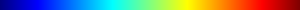Finally, FreeSurfer cannot read binary spreadsheets like this. The file has to be converted to simple values separated by space. In LibreOffice or OpenOffice, there is a very simple way of doing this. Go to File -> Save As… In the File type, choose Text CSV, and make sure to mark the option Edit Filter Settings: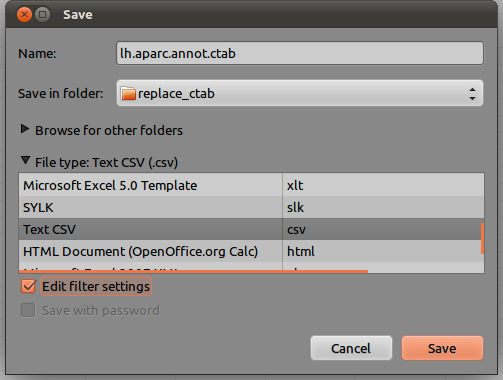On the next window, as Field Delimiter, choose {space}. As Text delimiter, leave blank, as shown below: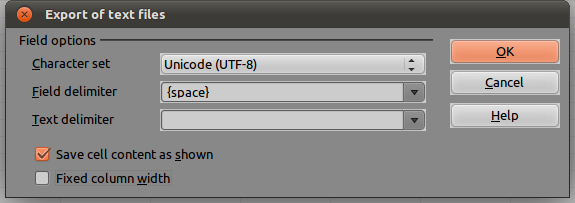The resulting file, saved to the disk, should look like this (click here for the full file):  0 bankssts 92 255 163 0 1 caudalanteriorcingulate 143 255 112 0 2 caudalmiddlefrontal 0 92 255 0 [...] 34 transversetemporal 255 133 0 0 35 unknown 160 160 160 0  ## 2. Embed the table into annotation file Once a table has been produced, it can be embedded into the annotation file that defines the the vertices that belong to each region. The annotation files produced by FreeSurfer already contain a default color table, which can be replaced. This can be accomplished by the Octave function replace_ctab.m. This function needs Octave 3.4.0+ or matlab. Type help replace_ctab at the prompt to get usage information. Here is an example: replace_ctab('${SUBJECTS_DIR}/bert/label/lh.aparc.annot',...
'${SUBJECTS_DIR}/bert/label/lh.aparc.annot.ctab.csv',... '${SUBJECTS_DIR}/bert/label/lh.aparc.annot.new');


In this example, the original annotation is stored in the file ${SUBJECTS_DIR}/bert/label/lh.aparc.annot, the new color table, as saved in LibreOffice, is in the file ${SUBJECTS_DIR}/bert/label/lh.aparc.annot.ctab. The new annotation file, with the new colortable, will be in the file ${SUBJECTS_DIR}/bert/label/lh.aparc.annot.new. Notice that for the function replace_ctab to work, the directory ${FREESURFER_HOME}/matlab must be in the Octave or matlab path.

## 3. Display with tksurfer

Once the new annotation file has been produced, display the result is straightforward:

tksurfer bert lh pial -annotation lh.aparc.annot.new


The result should be like the brain shown at the beginning of this article.

Update (01.Aug.2013): In more recent FS versions, the “lh” in front of the annotation file can be omitted, as it’s already specified in the same command line (thanks to Krishna Pancholi, Olin Neuropsychiatric Research Center, for the tip.). This affects only the last command line, which becomes then:

tksurfer bert lh pial -annotation aparc.annot.new